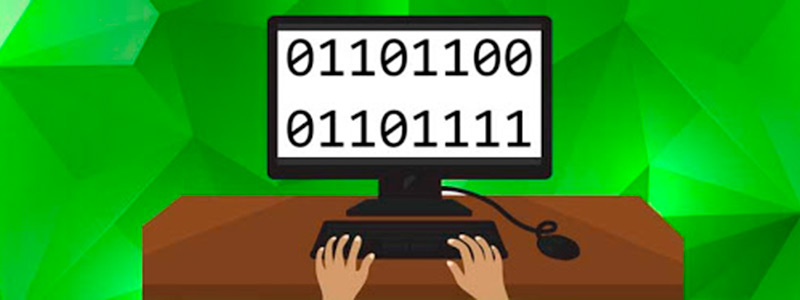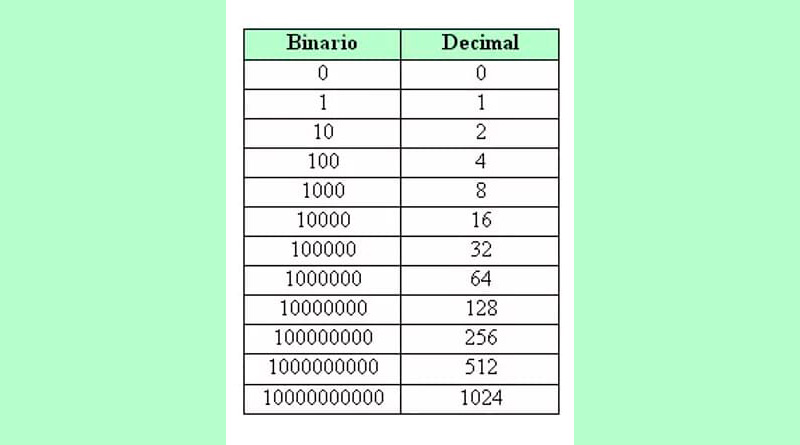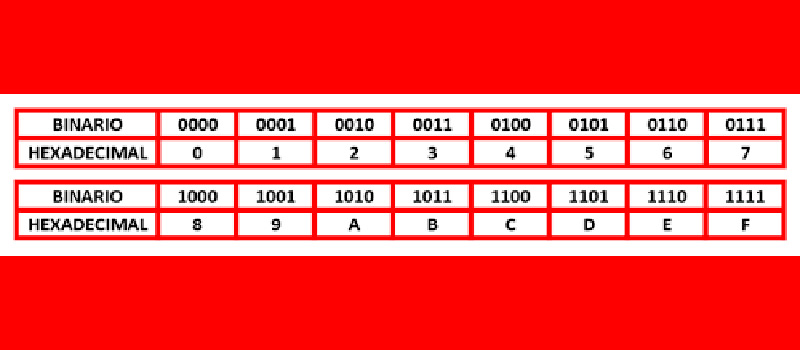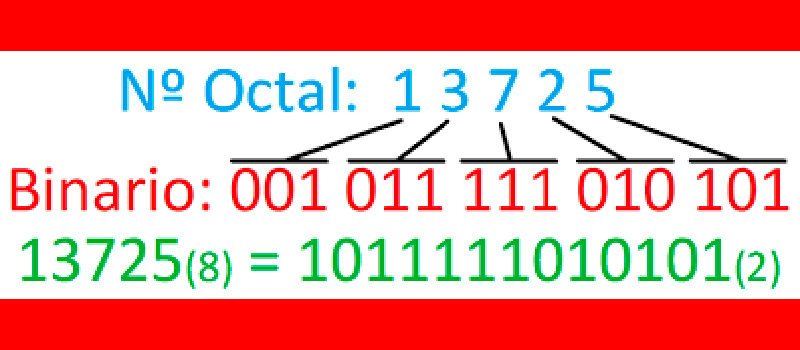BINARY SYSTEM  What is it? + History ▷ 2020

In mathematics there is a large complex set of number systems that have different bases and have distinctive characteristics between them. One of these is the binary system, which works with two numbers, “0” and “1”.

This system is very important for computing, since from this arises the term bit. Through electronic pulses and bits, software executes actions on a computer.

In the following paragraphs you will find the most complete information about this base 2 system. We will show you the necessary steps you must take to convert binary numbers into hexadecimal, octal and decimal.

What is the binary system and what is it used for in computing and computing?Binary numbers is a base 2 mathematical system, that is, the allowed values ​​are “0” and “1”. So there is a big difference with the decimal system that we use frequently in different aspects of our lives. In computer science the number zero and one are called binary digits and it is translated as “Binary digit” or commonly known as “bit”.

As far as you can tell the “Bit 0” and the “Bit 1” to represent electronic pulses, generally using 0 as a disconnect and 1 as a positive signal or connection. In this way, programs installed on a computer can represent computer instructions, read text or represent data.

This can be done through the work done by the microprocessors to detect the presence or not of the electrical pulse signal. Processors have a different transfer rate according to their manufacture and can group up to 8 bits into a single signal. This set is what is called “byte” y is the unit of measurement used in computing.

History and origin When is the binary system discovered and what is its impact?

The first appearance of the binary system appears through the mathematician Pingala, 3 centuries before the birth of Christ. But, others claim that there is a work in the Chinese oracular book I Chin whose creation dates back to 1200 BC and in which certain patterns with binary numbers are established. With this same prayer book, an arrangement was made for a decimal sequence that included the numbers 0 to 63.

This work was in charge of the philosopher Shao Yong and made it in the 11th century of our era. But it was not until 1605 that the chancellor of England, the philosopher and mathematician Francis Bacon, adopted a system in which it established that letters could be represented in binary numbers.

This led him, 70 years later, to publish the Spanish monk Juan Caramuel a more realistic description of this base 2 system. Already in the nineteenth century, what would be a paradigm break of the binary number system. A detailed logic was established for how these numbers should be treated. This was done by the British George Boole, which later became known as Boolean algebra.

In the late 1890s, the United States needed to do a census of its population, so it hired Herman Hollerith to do this job. The German used a binary system by means of punch cards based on Joseph Marie Jacquard, which performed a binary control for its looms. It is at this moment where binary numbers enter computing.

From that moment the information began to be processed automatically without the help of human beings. Years later, electrical engineer jobs appeared Claude Shannon and scientist George Stibitz. In these, the standards for the use of the binary system in computing began to be established.

Binary system vs decimal vs octagonal vs hexadecimal How is each different?

The binary system has its base 2, that is, the numbers used are the 0 and 1. On the other hand the decimal system its base is 10 and it is the system we use on a daily basis. While the system octagonal the base is 8 and the numbers are used 0, 1, 2, 3, 4, 5, 6, 7. Finally, the system hexadecimal has its base of 16 and is represented by the Arabic numerals and letters of the alphabet, which are 0, 1, 2, 3, 4, 5, 6, 7, 8, 9, A, B, C, D, E, F.

The binary system accepts negative or inverse integers0 being the reference point for the incorporation of the sign, but these numbers are represented in a particular way. While in the decimal system Whole and fractional numbers are accepted and can have positive and negative signs represented by a – in front of each number before zero.

Regarding the octal system accepts fractions and positive and negative numbers. Hexadecimal considers negative any number less than 7, so everything F that it is found as the first digit means it is negative and should not be calculated.

Converting Between Systems How do you migrate data from one to another?

To convert numbers from one system to another you will have to perform some steps, which we will show you below:

Binary and decimalTo convert a binary number you should start counting the digits starting from the right and the first being “0”. This way if you have the number 11011 you will count from the last “1” as the number 0. So your account will be like this:

• 1(4) 1(3) (2) 1(1) 1()> 4, 3, 2, 1, 0

Next, you will have to make a base power sum 2, being the exponents the numbers 4, 3, 2, 1, 0. To each adding you must multiply it by binary numbers. That is, by 1, 1, 0, 1, 1.

In this way, the conversion that would give:

• 24 + 1.23 + 0.22 + 1.21 + 1.1 =
• 16 + 8 + 0 + 2 + 0 =
• 26

That is, 11011 in binary system it is equal to the number 26 in a decimal system.

In case you want to convert from decimal to binary you will have to take the decimal number and divide it in two. Then to that result you will have to return it to divide into 2 and so on until finished.

If he the rest was 1, you should take it into account, since you will take all the quotients and expose them inversely to form the binary number.

For example, if you have the number 100 you will have to do the following:

• 100/2 = 50 the remainder is
• 50/2 = 25 the remainder is
• 25/2 = 12 the remainder is 1
• 12/2 = 6 the remainder is
• 6/2 = 3 the remainder is
• 3/2 = 1 the rest is 1

Therefore, the number binary what is formed is 1100100. This means that the number 100 in a decimal system is equal to the binary number that has been obtained.To convert a binary number to a number of the hexadecimal system you must group by groups of 4 digits. In case you have fewer digits you will have to complete with leading zeros.

Then you will have to put the numbers in each digit 8, 4, 2, 1. Next, you will need to multiply those numbers by each digit and add them. For example, if you have a binary number 1010 you must do the following: 8 x 1 + 4 x 0 + 2 x 1 + 1 x 0 = 10 and since 10 is greater than 9 the number corresponds TO.

Therefore, the binary number 1010 represents the number A in the hexadecimal system. If you have larger digits you will have to do the same steps, but for each group of four digits.

That is, if you had the number 1100101101 you should do the following:

• Separate into four digits 11 0010 1101.
• Complete the first group with 0 until you can have the group of 4. That is, you will have 0011 0010 1101.

Perform the previous steps by multiplying and adding each group:

• 8 x + 4 x + 2 x 1 + 1 x 1 = 3

• 8 x + 4 x + 2 x 1 + 1 x = 2

• 8 x 1 + 4 x 1 + 2 x + 1 x 1 = 13> greater than 9, so the number D corresponds

This implies that the binary number 1100101101 is the same in hexadecimal system as 32D.

In case you want convert a hexadecimal number to binary you will have to directly replace the digit with its corresponding binary number.

For that you will have to take into account the following relationship:

• 0 = 0000
• 1 = 0001
• 2 = 0010
• 3 = 0011
• 4 = 0100
• 5 = 0101
• 6 = 0110
• 7 = 0111
• 8 = 1000
• 9 = 1001
• A = 1010
• B = 1011
• C = 1100
• D = 1101
• E = 1110
• F = 1111

If we do it with the previous case 32D, we will have to replace each digit.

So that:

• 3 = 0011
• 2 = 0010
• D = 1101

In this way the binary number is formed 001100101101, or what is the same 1100101101. That is, the number that was formed is the same that we took to convert it into the step of binary to hexadecimal.

Binary and octagonalAs with the conversion to a hexadecimal system you will have to group the numbers, but in this case in groups of three digits. You will also have to complete with 0 to the left when they do not reach a group of 3 digits.

In addition, you should keep in mind the following replacement table, with the first binary digits and the second corresponding to an octal system:

• 0 = 0
• 001 = 1
• 010 = 2
• 011 = 3
• 100 = 4
• 101 = 5
• 110 = 6
• 111 = 7

What you should do now is group then replace the numbers by means of the table, for example, if you have the number 1011101.

You will have to follow this process:

• Do the groups of 3 digits, that is, you will have 1 011 101 left.
• Now, complete with 0 the first group, you will have as a result 001 011 101.

Replace according to list that we show you before.

You will have:

• 001 = 1
• 011 = 3
• 101 = 5

Therefore, the binary number 10111012 corresponds to a system octagonal to the number 1358. In case you want turn into a number from octagonal to binary system you will have to follow the path reverse. That is, you will have to replace each digit you have of the octal system by groups of three digits of the binaries. You shall keep in mind the list that we mentioned before.

So if you have a number 24578 and you want to convert it to binary, you will have to separate it into 2, 4, 5, 7.

Next, you will need to follow the replacements for each number:

• 2 = 010
• 4 = 100
• 5 = 101
• 7 = 111

In this way it is concluded that the number 24578 = 101001011112

If you have any questions, leave them in the comments, we will answer you as soon as possible, and it will surely be of great help to more members of the community. Thank you! 😉

Rate this post